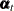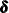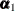The SSM Procedure

PROC SSM Statement

• PROC SSM <options>;

The PROC SSM statement is required. You can specify the following options in the PROC SSM statement:

DATA=SAS-data-set

specifies the name of the SAS data set that contains the variables needed for the analysis. If you do not specify this option, PROC SSM uses the most recently created SAS data set.

NOPRINT

turns off all the printing and plotting for the procedure. Any subsequent print options are ignored.

PLOTS <(global-plot-options)> = plot-request <(options)>
PLOTS<(global-plot-options)> = ( plot-request <(options)> <... plot-request <(options)> > )

controls the plots produced with ODS Graphics. When you specify only one plot-request, you can omit the parentheses around it. Here are some examples:

plots=none
plots=all
plots=residual
plots=residual(normal)
plots=(maxshock residual(normal))
plots(unpack)=residual


If you do not specify any specific plot-request, then by default PROC SSM produces the plot of standardized residuals against time. For general information about ODS Graphics, see Chapter 21: Statistical Graphics Using ODS in SAS/STAT 13.2 User's Guide.

Global Plot Options:The global-plot-options apply to all relevant plots generated by the SSM procedure. The following global-plot-option is supported:

UNPACK

displays each graph separately. (By default, some graphs can appear together in a single panel.)

Specific Plot Options:The following list describes the specific plot-requests and their options:

ALL

produces all plots appropriate for the particular analysis.

AO< (prediction-error-plot-options)>

produces the prediction error plots—one for each response variable. You can specify the following prediction-error-plot-options:

NORMAL

produces a summary panel of the prediction error diagnostics, which consist of the following:

• histogram of prediction errors

• normal quantile plot of prediction errors

STD

produces a scatter plot of standardized prediction errors against time.

MAXSHOCK

produces a scatter plot of maximal state shock statistics against time.

NONE

suppresses all plots.

RESIDUAL <(residual-plot-options)>

produces the residuals plots—one for each response variable. You can specify the following residual-plot-options:

NORMAL

produces a summary panel of the residual diagnostics, which consist of the following:

• histogram of residuals

• normal quantile plot of residuals

STD

produces a scatter plot of standardized residuals against time.

See the section Delete-One Cross Validation and Structural Breaks for more information about the precise meaning of the terms maximal state shock statistics and prediction errors.

PRINTALL

turns on all the printing options for the procedure. All subsequent NOPRINT options in the procedure are ignored.

STATEINFO

prints two tables that provide information about the composition of the state vector in terms of the components specified in the model. One table describes the composition of state, and the other table describes the diffuse vectorand the regressors, which are part of the initial condition specification. See the section State Space Model and Notation for more information about the state space model notation.

OPTIMIZER( <TECHNIQUE=technique> <MAXITER=integer>)

specifies options that are associated with the optimizer used in the maximum likelihood parameter estimation. The default settings of the optimization process are adequate in most problems. However, in some cases it might be useful to change the optimization technique or to change the maximum number of iterations. You can specify one of the following techniques:

ACTIVESET

corresponds to the active-set method.

DBLDOG

corresponds to the double-dogleg method.

INTERIORPOINT

corresponds to the primal-dual interior point method.

NEWRAP

corresponds to the Newton-Raphson method.

QUANEW

corresponds to the (dual) quasi-Newton method.

TRUREG

corresponds to the trust region method.

The default technique is TRUREG. The INTERIORPOINT and ACTIVESET techniques are documented in Chapter 10: The Nonlinear Programming Solver in SAS/OR 13.2 User's Guide: Mathematical Programming and the remaining techniques are documented in Chapter 6: Nonlinear Optimization Methods. You can alter the maximum number of iterations setting in the nonlinear optimization search by specifying a nonnegative integer as the MAXITER= value.